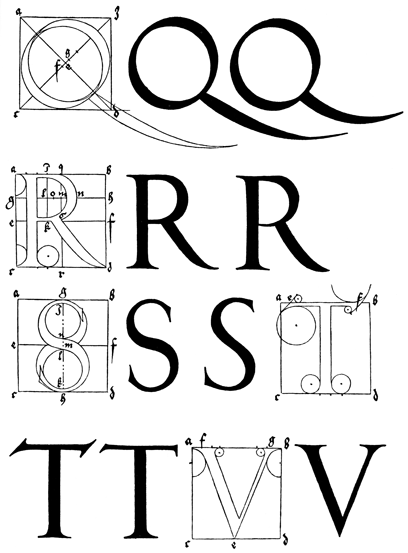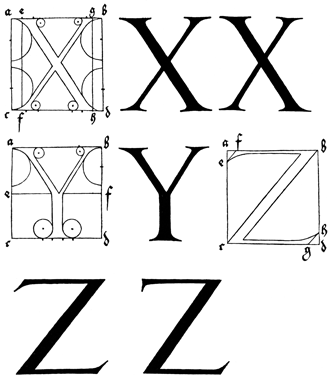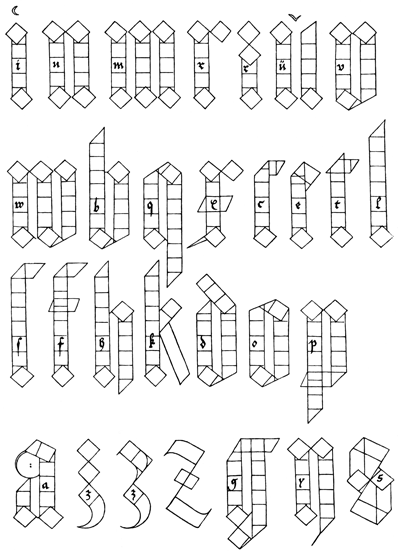# “Directions for the Construction of the Text” — Albrecht Dürer“Directions for the Construction of the Text” —  Albrecht Dürer

(From  Of the  Just Shaping of Letters).

THE letters which are usually called “text,” or quadrate, it was formerly customary so to write, although they are now imitated by the new art, as presently I shall show below. Although the alphabet begins with the writing of A, yet shall I (not needlessly) in the first place undertake to draw an I; because almost all the other letters are formed after this letter, although always something has to be added to it or taken away.

First make your I of equal squares, of which three are properly set one over the other; and the top of the top one, and the bottom of the bottom one, divide in two points, that is to say, into three equal parts: then set a square equal to the others in an oblique manner, so that its diagonal be vertical, and its angle on the first point of the top square. In this way, this oblique square shall extend with its angles more to the left than the right. Then produce upwards on either side, after the width of the superposed squares, right lines to meet the sides of the oblique set square. Next do below precisely as you did above, except that you must set the angle of the oblique square on the second point, that is, the one farthest to the right in the bottom of the lowest square; and let fall your lines on either side upon the transposed square: so will I be perfect; only above it draw with a fine pen a tiny in-crescent.

So shall you make N from two standards of this same I, set so that their angles at top and bottom touch; and in this manner the space between the two shall be narrower than the breadth of either: also, you shall no longer put little crescents above them; you must make of the same length all the short letters throughout the alphabet.

In like manner make M of three standards, just as you made N of two.

R make as I, except only that at top you must set an equal square diagonally, to the right, so that angle touch angle. R you may make also in this fashion: below leave its foot as before, but above add two diagonal squares, which shall touch each other with their angles in the middle point of the vertical limb, and then produce upwards both sides of the latter to meet the diagonal square.

V is made in three ways. First let it be made simply, as N; only that in the farther limb, you shall omit at the top the diagonal square; and instead shall draw an oblique line, so that it may make two angles in this limb (produced) of which the farther shall be of the same height as that of the topmost angle of the diagonal square, & the higher angle of the same height as the angle nearest to it in the said square.

The second V, which we use at the beginning of a sentence, make thus: Draw the first limb as before for I; only, at the bottom, push the diagonal square a little further to the right, so that its hither angle does not project beyond the side of the vertical limb, but falls in the line of its descent. Then set the second limb to the right of this, and cut it off below by an oblique line, drawn from the lowest angle upwards to the right, as far as the middle point of the lowest of the three superposed squares.

Next make W (i. e., double V) just as you made V-simplex; only you shall set before it the standard limb of I.

B make as the second V-simplex; but in the first upright omit the diagonal square at the top, and set upon the three original squares three others similar, but the seventh one you shall cut off diagonally from its lower hither angle.

Likewise when your B so made is turned upside down, then it will be a Q.

X you shall construct from I. Append from top angle to the right a diagonally set square, as you did before in R; and at the bottom draw an acute tail to the left from the diagonal, and at the middle of the vertical limb describe a transverse, in such way that the former is cut before and aft by the latter’s diagonal; let the hither and lower angle be terminated as far in front of the upright as would measure one-half of the cutting diagonal, which at top shall just touch the upright; but to the right let the transverse at top project to a point just below the angle of the oblique square; from thence downwards let it be cut off by an oblique line parallel to the anterior diagonal.

C you shall construct from I after this fashion: Remove the top diagonal square, & let lines be produced on either side to the proper height of the letter, and cut off the hither angle by a diagonal; then draw at top a broad transverse, projecting beyond the vertical to the right the width of the latter, and cut this off by a diagonal in such a way that it project below only half as far as above.

The vertical standard of E you shall make as for C; but from above let there descend to the right a broad limb from the diagonal bisecting the right angles of one square, and one-third again as long as broad; and let there be drawn from its lower angle a small diagonal line to the vertical limb.

T shall be made like C, except that at top something is added to its diagonal, so that its tip converges to a fine point, and the like to the left on the hither side of the broad standard, just as at the top: and because of this is T at top more elegant than C, and has not the same incurved appearance.

L you are to make below like I; only six squares are to be set on end; then cut off the hither side of the seventh by a diagonal, and so the apex of the letter shall remain to the right.

The letter S you shall make as L; except that at top to the right must be drawn a broad limb of the length of the diagonal, which afterwards you are to cut off by a line parallel to the diagonal.

F you shall make as S, just adding to it a transverse limb at the height of the shorter letters and double as long as broad, so that the point on the hither side & below shall project as far as half the limb’s breadth, so that the two diagonal abscissions may be equidistant from one another.

The near limb of the letter H make like L, and to it join by its top, in the proper place, the farther made like I; but below, for the diagonal square, substitute a fourth square in line with the others, and the fifth and lowest cut off on the far side by its diagonal.

Of K make the near limb like L; and to the right of it append a diagonal square, from the lowest angle of which let a line be obliquely produced to meet the said vertical limb; and next from this line let a broad limb be obliquely drawn, and this, at the bottom, you are to cut off by a diagonal, in such fashion that the space below, between the two tips shall not be more than the diagonal of a single square.

D in its lower half make like B; but at top let the anterior limb ascend upwards to the maximum height of the letters, and then cut off the hither angle by its diagonal; next superpose to the same height half a square upon the other three squares of the farther limb, & once more do here as you did below, and let this broken limb rest on the angle of the near limb, and let it extend beyond it as far as the end of the upright near limb; and so will it all but contain three conjunct squares; for when it meets the near vertical limb, that fraction is to be cut off at right angles.

O you are to make below as D, and also the same at the top as the bottom, only, as it were, turning it upside down.

The anterior limb of P make like L inverted; but the posterior like the standard of I: at bottom, however, you are not to add an oblique square, but amputate the limb diagonally, & draw at the bottom a broad transverse limb, which likewise is to be cut off diagonally, so that the lower point shall project to the left, a distance of half the breadth of the limb.

Likewise A in the lower half you are to make like N; but of its anterior vertical limb, you are to cut off the hither angle of the middle square by its diagonal; of the posterior, however, allow three squares to remain superposed, and incline the top part (the fourth square) rather to the left, so that if at this side is joined to it the half of a square, then it shall attain the height of the letter; and cut off the square obliquely, yet so that the lower point shall project farther than the upper; then describe to the left a circle, sweeping downwards, so that its contents shall embrace the farthest limit of the anterior limb.

Z is made in threefold fashion. First set a diagonal square which shall touch the height of the letter; then add another like it, on the right, joining their sides, & let these form a quadrangle sloping downwards on the right: next set a diagonal square in straight line under the top square, and distant from the lower one the length of its diameter: then draw a diagonal line between the near angles of these two squares, or make a rounded limb to reach the lower square; but from the said lowest square of all you shall draw downwards and to the right, by the aid of divers circles, a round extension, whose bottom shall mark the length of the letter; and let its tip, sharp and tenuous, verge to the left. Or construct Z of three oblique limbs, one above the other, & to connect them draw the diagonal, which shall slope upwards to the right.

Another Z you may make in this way: Let three diagonal squares be set atop of one another; and let the lowest have a rounded extension, as in the first Z.

The first limb of G make below like I, and add at the bottom another diagonal square, joining the two by their angles; but at top produce the farther tip of this limb upwards to the height of the letter, & from this point draw a diagonal downward to the left, as far as the hither angle of the first right square of the three set one on other. Next draw the farther vertical standard entire, of the same length as the hither standard, and at the bottom draw a diagonal from the angle of the lowest oblique square to touch the tip of the angle of the farther limb, & on the inner side produce downwards the side of the limb, to meet the tip of the said diagonal; to this also, by one line, join the lowest of the hither squares. Now draw at top a transverse limb of the customary breadth, from the back of the nearer vertical limb, passing through the farther one, and reaching as far beyond this as its breadth; & this limb, finally, you shall cut off by an oblique line parallel to that of the near limb.

Y you shall make as N, only at bottom must be omitted the farther diagonal square, & in its place is to be set a right square under the other three superposed squares; then split the fifth square by a diagonal, so that the tip shall be in front; from which let there be produced a diagonal line, equal in length to a single side of the square.

Curved, or short S, you shall make on this wise. At the middle height of the letter, let there be set, close to one another, their angles touching, two oblique squares; from the near square draw a broad vertical limb to the height of the letter; and in the same fashion, from the farther square let one fall downwards—just as you constructed I top & bottom. Next cut off both these limbs, one at top and one at bottom, by diagonals, in such fashion that the sharp tips of both may be on the side near the middle. Then let there be drawn two broad limbs—namely, from the upper, to the right, and downwards; and in like manner, from the lower, upwards, and to the left; of the breadth of the limb, above and below, but let them be produced no further than the breadth of the distance between the limbs: then draw a diagonal downwards, from right to left, which shall cut off both oblique limbs. To it also you must produce the sides of the squares set in the midst.

So, accordingly, have I set them down—in skeleton in rotation, and in proper order in black. This (as I said above) is the antique form of the letters; but in these days there is used a more elegant text, and a diagonal square is substituted in the middle place for a right square, so that the lines of the letters are not so much curved; and there are made certain limbs adjoined and cleft; and there are set one on another three squares & a half; and spaces are left between two limbs as great as their width. Letters of this sort also have I set forth on the third page following; as also capital letters, which are called “versals,” because they are customarily set at the beginning of a verse; and these ought to be made one-third higher than the remaining shorter letters in writing.Albrecht Dürer

## 3 thoughts on ““Directions for the Construction of the Text” — Albrecht Dürer”

This site uses Akismet to reduce spam. Learn how your comment data is processed.